# Improper Integrals

Also found in: Dictionary.

## Improper Integrals

a generalization of the classical concept of the integral for the case of unbounded functions or functions defined on an infinite interval of integration. The definite integral as the limit of Riemann sums can exist (can have a definite finite value) only for bounded functions defined on a finite interval. Therefore, if the interval of integration or the integrand is not bounded, then still another limiting process is required in order to define the integral. Integrals thus obtained are called improper integrals.

If a function f (x) is integrable on any finite interval [a, N] and if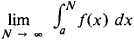exists, then the latter is said to be the improper integral of f(x) on the interval [a, ∞] and is denoted by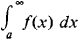In this case, the improper integral is said to converge. When this limit and thus also the improper integral do not exist, then the improper integral is said to diverge. For example,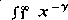dx converges for γ > 1 and diverges for γ ≤ 1. Improper integrals are similarly defined on the intervals [-∞ ,b] and [—∞ ,∞].

If a function f(x), defined on an interval [a, b], is not bounded in a neighborhood of the point a but is integrable on any interval [a + ∊, b], 0 < e < b − a, and if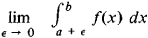exists, then this limit is called the improper integral of f(x) on [a, b] and is usually written as an ordinary integral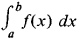We proceed in a similar manner if f(x) is not bounded in a neighborhood of the point b.

If the improper integral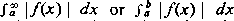exists, then it is said that the improper integralis absolutely convergent; if the latter integrals, however, converge while the former diverge, then the improper integral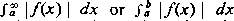is said to be conditionally convergent.

Problems leading to improper integrals were examined in geometrical form by E. Torricelli and P. de Fermat in 1644. Precise definitions of improper integrals were given by A. Cauchy in 1823. The distinction between conditionally and absolutely convergent integrals was made by G. Stokes and P. G. L. Dirichlet (1854). A number of mathematical works in the 19th century were devoted to the evaluation of improper integrals in cases where the corresponding antiderivatives could not be expressed in terms of elementary functions. The basic methods of evaluating improper integrals are differentiation, integration with respect to a parameter, series expansion, and application of the theory of residues. The values of many improper integrals are given in various tables.

Improper integrals are very important in many areas of mathematical analysis and in applications. In the theory of special functions (cylindrical functions, orthogonal polynomials), one of the fundamental research methods is the representation of the functions in the form of improper integrals depending on a parameter. A relevant example is the gamma function, ┌ (ɑ) =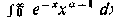. Improper integrals include the Fourier integral, as well as the integrals encountered in other integral transforms. Solutions of boundary-value problems in mathematical physics are written as multiple improper integrals with an unbounded function as integrand. The improper integral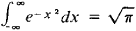is very important in probability theory, as is the improper integral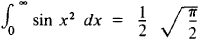in the theory of the diffraction of light.

In a number of cases, a definite value may be assigned to a divergent improper integral. In particular, if the integral f(x) dx diverges but the symmetric limit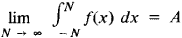exists, then A is called the Cauchy principal value of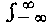f(x) dx. Thus, the Cauchy principal value of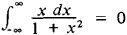is 0. The Cauchy principal value of an improper integral of an unbounded function is similarly defined. A theory of integral equations involving improper integrals understood in the Cauchy principal value sense has been constructed by N. I. Muskhelishvili and his students.

### REFERENCES

Smirnov, V. I. Kurs vysshei matematiki, 20th ed., vol. 2. Moscow-Leningrad, 1967.
Fikhtengol’ts, G. M. Kurs differentsial’nogo i integral’nogo ischisleniia, 7th ed., vol. 2. Moscow, 1969.
Kudriavtsev, L. D. Matematicheskii analiz, vol. 1. Moscow, 1970.
References in periodicals archive ?
for t [greater than or equal to] T and the improper integral of the larger function converges for [absolute value of v] < k, then by the comparison test, the integral [MATHEMATICAL EXPRESSION NOT REPRODUCIBLE IN ASCII] converges for [absolute value of v] < k.
1] given by the improper integrals (10) and (11) satisfy:
approaches a finite limit as A [right arrow] [infinity], then we call that limit the improper integral of first kind of f from a to[infinity]and write
In contrast with the usual convolution algorithm, the nonhistory-dependent algorithm described in the previous section needs the replacement of the improper integral by a finite sum.

Site: Follow: Share:
Open / Close